# 978-0073382395 Chapter 10 Questions and Problems 30-36

Document Type
Homework Help
Book Title
Fundamentals of Corporate Finance Standard Edition 9th Edition
Authors
Stephen Ross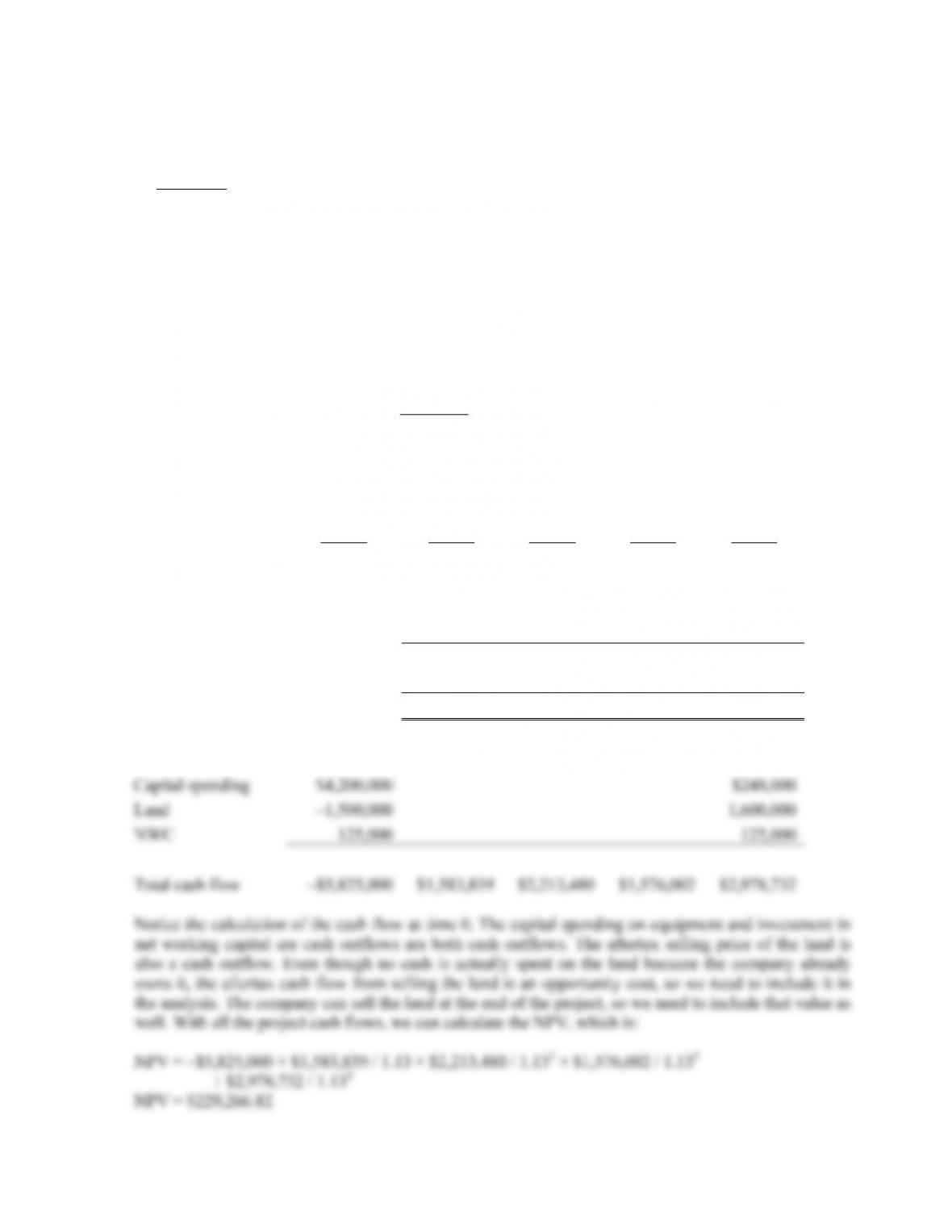B-192 SOLUTIONS
30. Surprise! You should definitely upgrade the truck. Here’s why. At 10 mpg, the truck burns 12,000 / 10 =
1,200
Challenge
31. We will begin by calculating the aftertax salvage value of the equipment at the end of the project’s life.
The aftertax salvage value is the market value of the equipment minus any taxes paid (or refunded), so
the aftertax salvage value in four years will be:
Taxes on salvage value = (BV – MV)tC
Taxes on salvage value = (\$0 – 400,000)(.38)
Taxes on salvage value = –\$152,000
Market price \$400,000
Tax on sale –152,000
Aftertax salvage value \$248,000
Now we need to calculate the operating cash flow each year. Using the bottom up approach to
calculating operating cash flow, we find:
Year 0 Year 1 Year 2 Year 3 Year 4
Revenues \$2,496,000 \$3,354,000 \$3,042,000 \$2,184,000
Fixed costs 425,000 425,000 425,000 425,000
Variable costs 374,400 503,100 456,300 327,600
Depreciation 1,399,860 1,866,900 622,020 311,220
EBT \$296,740 \$559,000 \$1,538,680 \$1,120,180
Taxes 112,761 212,420 584,698 425,668
Net income \$183,979 \$346,580 \$953,982 \$694,512
OCF \$1,583,839 \$2,213,480 \$1,576,002 \$1,005,732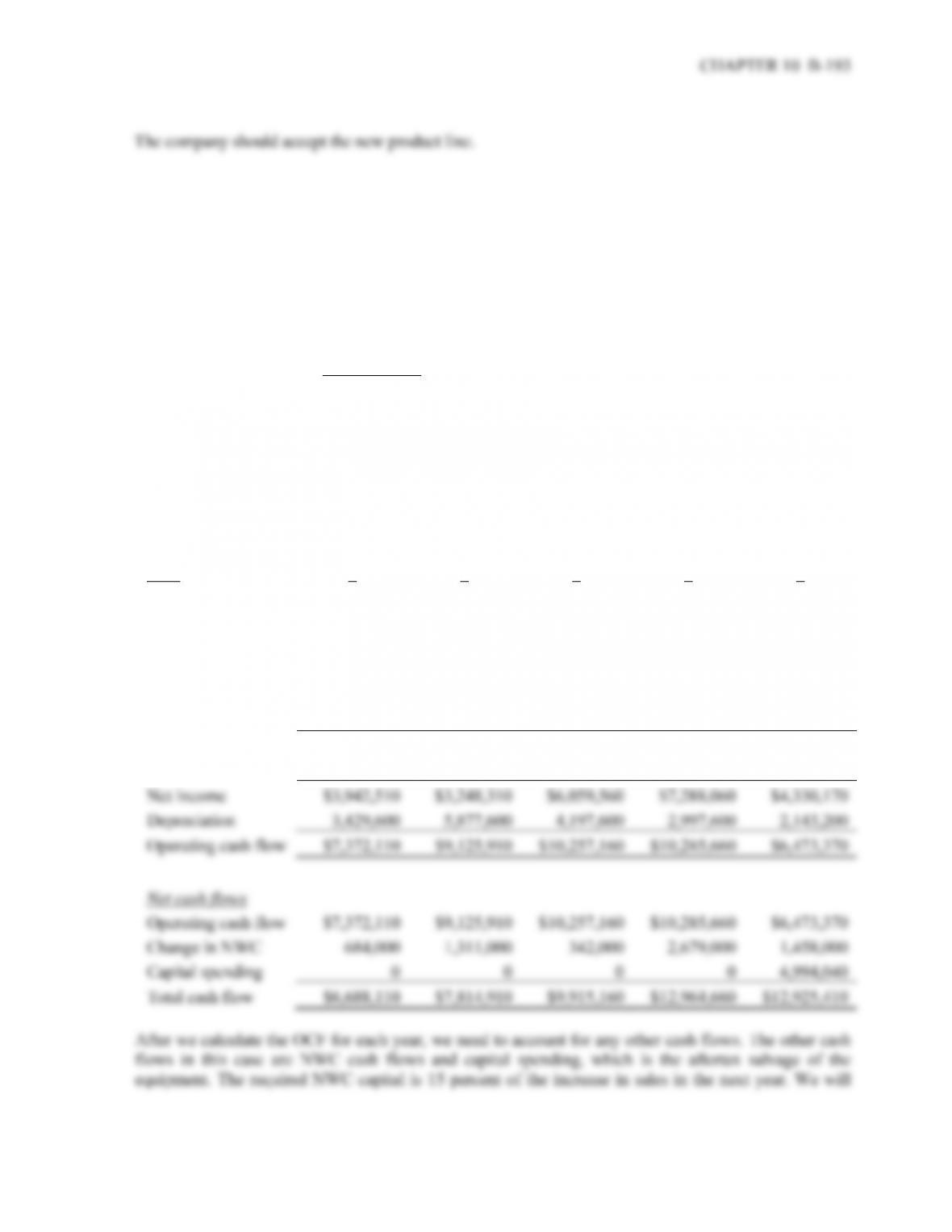32. This is an in-depth capital budgeting problem. Probably the easiest OCF calculation for this problem is
the bottom up approach, so we will construct an income statement for each year. Beginning with the
initial cash flow at time zero, the project will require an investment in equipment. The project will also
require an investment in NWC. The initial NWC investment is given, and the subsequent NWC
investment will be 15 percent of the next year’s sales. In this case, it will be Year 1 sales. Realizing we
need Year 1 sales to calculate the required NWC capital at time 0, we find that Year 1 sales will be
\$35,340,000. So, the cash flow required for the project today will be:
Capital spending –\$24,000,000
Initial NWC –1,800,000
Total cash flow –\$25,800,000
Now we can begin the remaining calculations. Sales figures are given for each year, along with the price
per unit. The variable costs per unit are used to calculate total variable costs, and fixed costs are given at
\$1,200,000 per year. To calculate depreciation each year, we use the initial equipment cost of \$24
million, times the appropriate MACRS depreciation each year. The remainder of each income statement
is calculated below. Notice at the bottom of the income statement we added back depreciation to get the
OCF for each year. The section labeled “Net cash flows” will be discussed below:
Year 1 2 3 4 5
Ending book value \$20,570,400 \$14,692,800 \$10,495,200 \$7,497,600 \$5,354,400
Sales \$35,340,000 \$39,900,000 \$48,640,000 \$50,920,000 \$33,060,000
Variable costs 24,645,000 27,825,000 33,920,000 35,510,000 23,055,000
Fixed costs 1,200,000 1,200,000 1,200,000 1,200,000 1,200,000
Depreciation 3,429,600 5,877,600 4,197,600 2,997,600 2,143,200
EBIT \$6,065,400 \$4,997,400 \$9,322,400 \$11,212,400 \$6,661,800
Taxes 2,122,890 1,749,090 3,262,840 3,924,340 2,331,630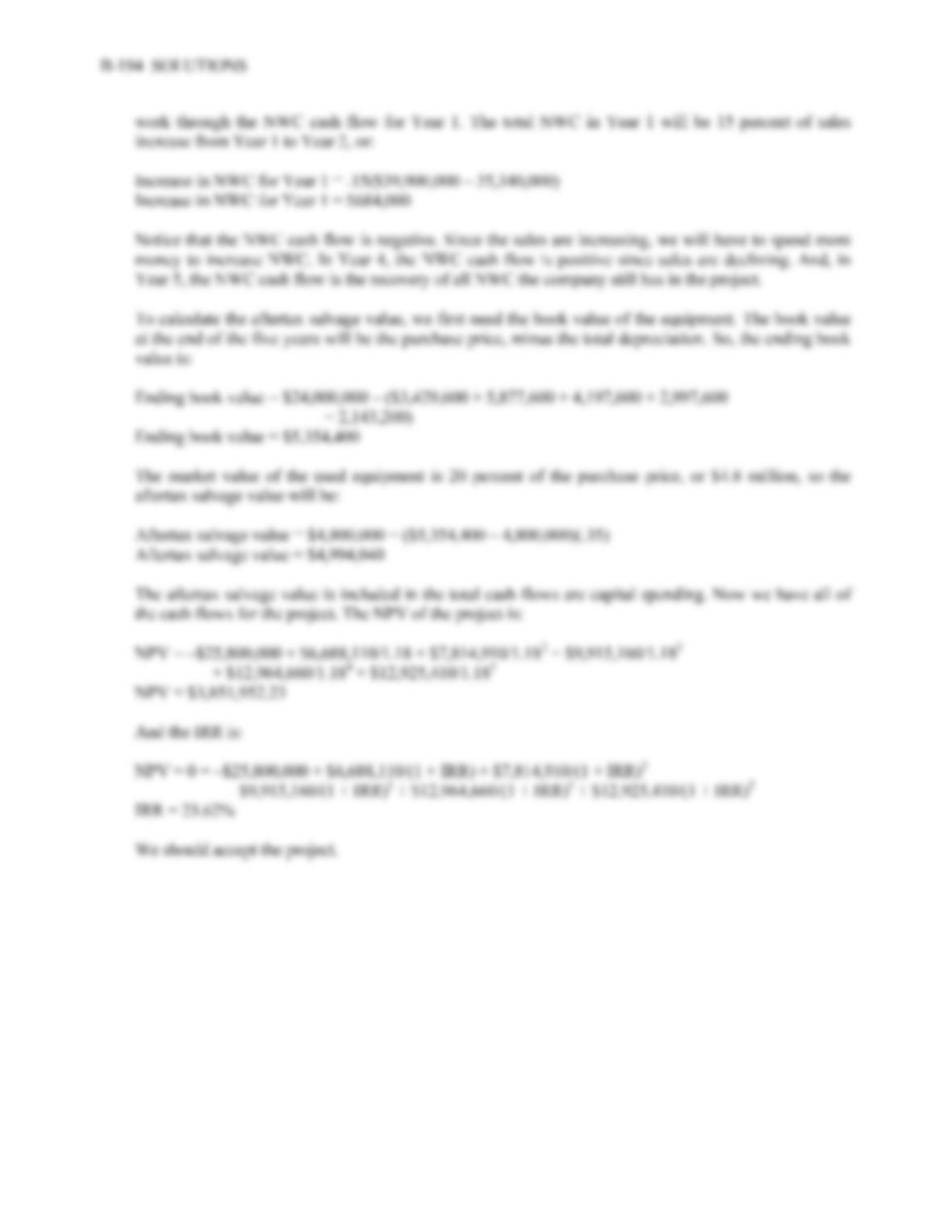33. To find the initial pretax cost savings necessary to buy the new machine, we should use the tax shield
approach to find the OCF. We begin by calculating the depreciation each year using the MACRS
depreciation schedule. The depreciation each year is:
D
1 = \$610,000(0.3333) = \$203,313
D
2 = \$610,000(0.4444) = \$271,145
D
3 = \$610,000(0.1482) = \$90,341
D
4 = \$610,000(0.0741) = \$45,201
Using the tax shield approach, the OCF each year is:
OCF1 = (S – C)(1 – 0.35) + 0.35(\$203,313)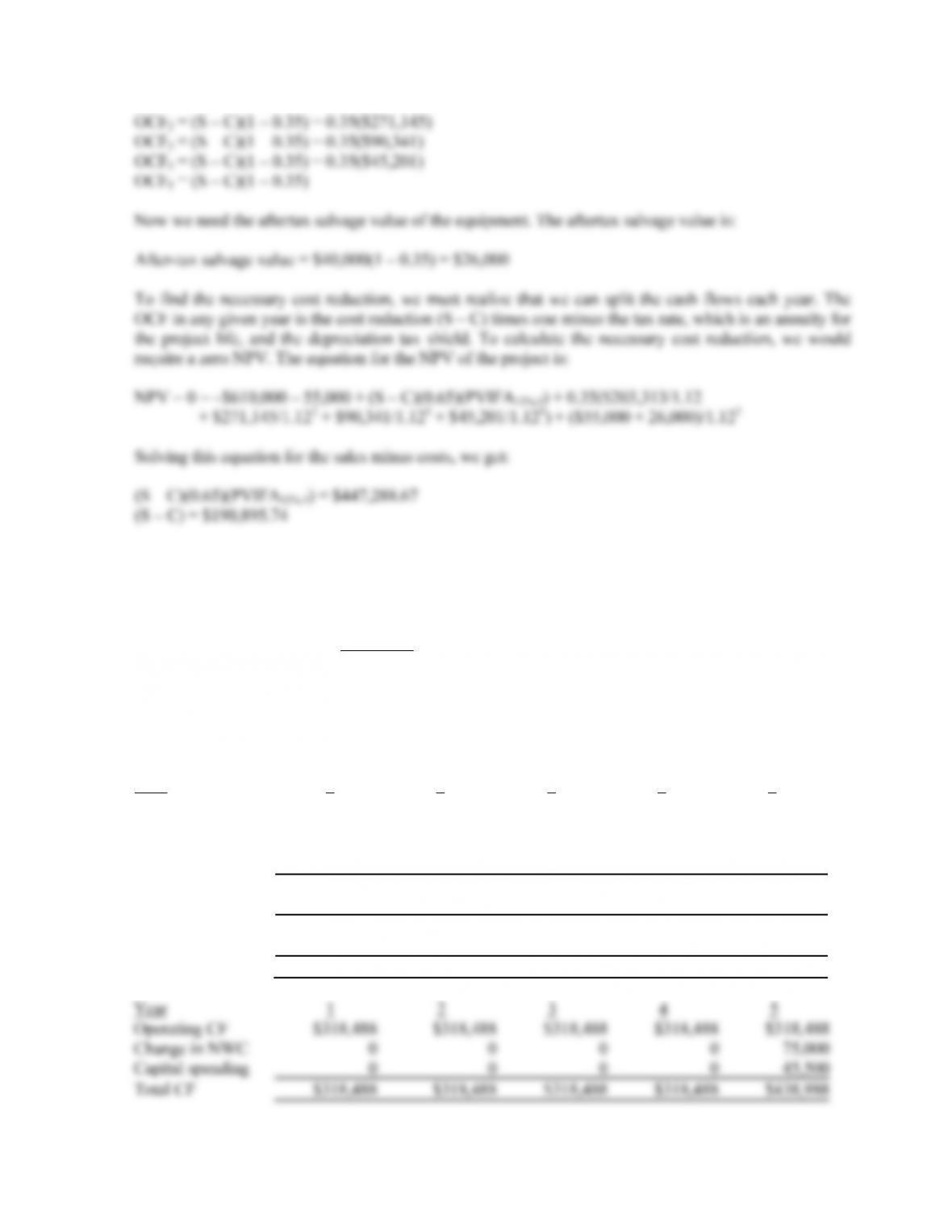CHAPTER 10 B-195
34. a. This problem is basically the same as Problem 18, except we are given a sales price. The cash
flow at Time 0 for all three parts of this question will be:
Capital spending –\$940,000
Change in NWC –75,000
Total cash flow –\$1,015,000
We will use the initial cash flow and the salvage value we already found in that problem. Using the
bottom up approach to calculating the OCF, we get:
Assume price per unit = \$13 and units/year = 185,000
Year 1 2 3 4 5
Sales \$2,405,000 \$2,405,000 \$2,405,000 \$2,405,000 \$2,405,000
Variable costs 1,711,250 1,711,250 1,711,250 1,711,250 1,711,250
Fixed costs 305,000 305,000 305,000 305,000 305,000
Depreciation 188,000 188,000 188,000 188,000 188,000
EBIT 200,750 200,750 200,750 200,750 200,750
Taxes (35%) 70,263 70,263 70,263 70,263 70,263
Net Income 130,488 130,488 130,488 130,488 130,488
Depreciation 188,000 188,000 188,000 188,000 188,000
Operating CF \$318,488 \$318,488 \$318,488 \$318,488 \$318,488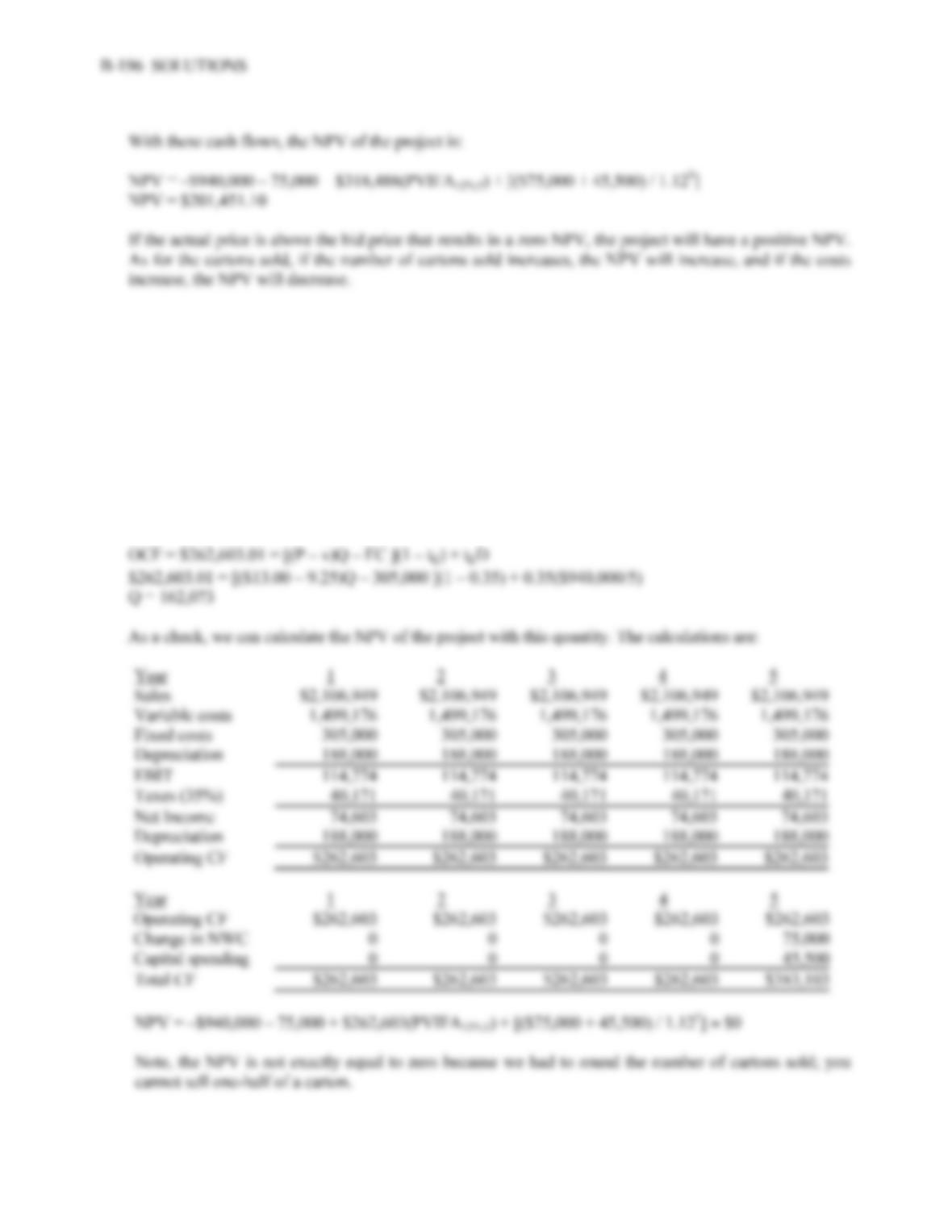b. To find the minimum number of cartons sold to still breakeven, we need to use the tax shield approach
to calculating OCF, and solve the problem similar to finding a bid price. Using the initial cash flow and
salvage value we already calculated, the equation for a zero NPV of the project is:
NPV = 0 = –\$940,000 – 75,000 + OCF(PVIFA12%,5) + [(\$75,000 + 45,500) / 1.125]
So, the necessary OCF for a zero NPV is:
OCF = \$946,625.06 / PVIFA12%,5 = \$262,603.01
Now we can use the tax shield approach to solve for the minimum quantity as follows: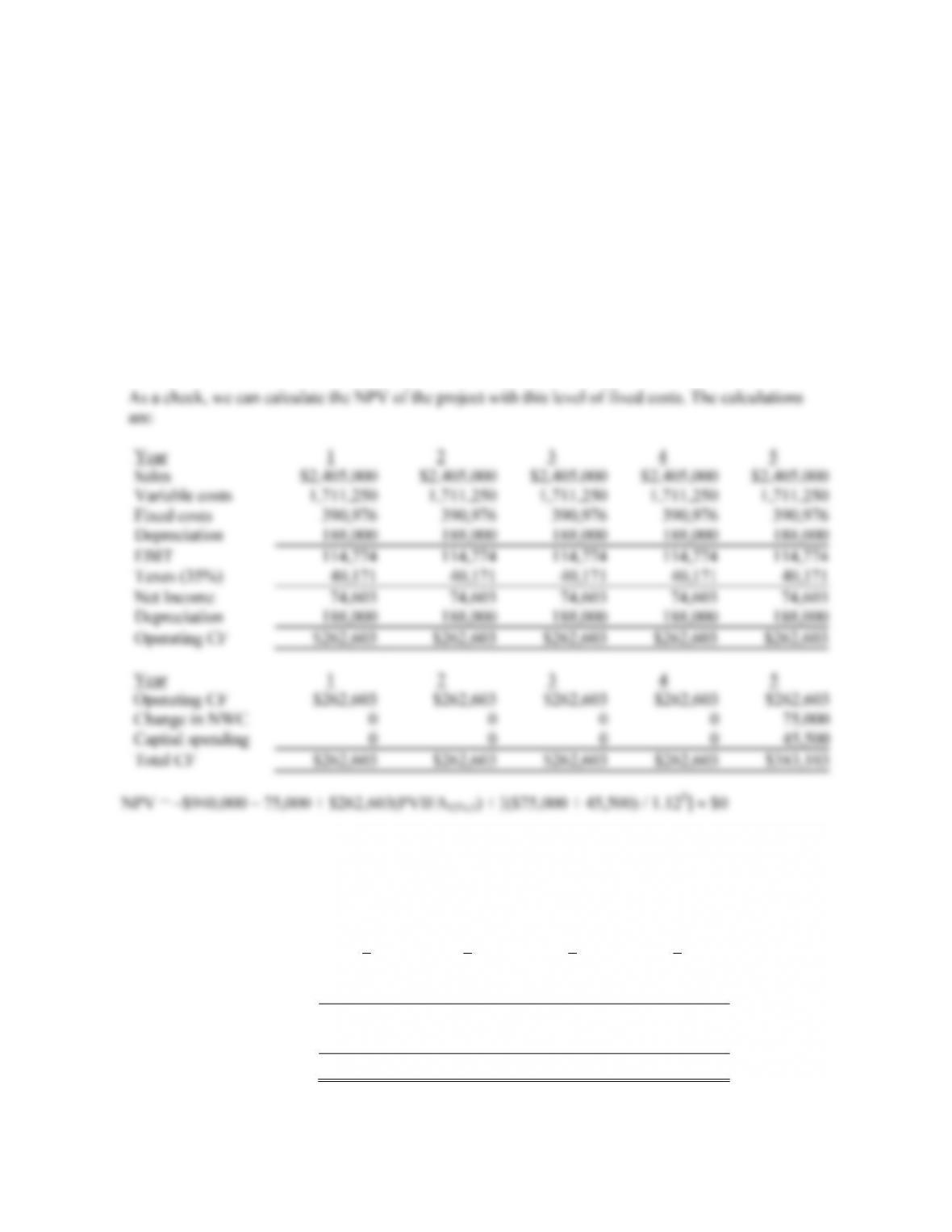CHAPTER 10 B-197
c. To find the highest level of fixed costs and still breakeven, we need to use the tax shield approach to
calculating OCF, and solve the problem similar to finding a bid price. Using the initial cash flow and
salvage value we already calculated, the equation for a zero NPV of the project is:
NPV = 0 = –\$940,000 – 75,000 + OCF(PVIFA12%,5) + [(\$75,000 + 45,500) / 1.125]
OCF = \$946,625.06 / PVIFA12%,5 = \$262,603.01
Notice this is the same OCF we calculated in part b. Now we can use the tax shield approach to solve
for the maximum level of fixed costs as follows:
OCF = \$262,603.01 = [(P–v)Q – FC ](1 – tC) + tCD
\$262,603.01 = [(\$13.00 – 9.25)(185,000) – FC](1 – 0.35) + 0.35(\$940,000/5)
FC = \$390,976.15
35. We need to find the bid price for a project, but the project has extra cash flows. Since we don’t already
produce the keyboard, the sales of the keyboard outside the contract are relevant cash flows. Since we
know the extra sales number and price, we can calculate the cash flows generated by these sales. The
cash flow generated from the sale of the keyboard outside the contract is:
1 2 3 4
Sales \$855,000 \$1,710,000 \$2,280,000 \$1,425,000
Variable costs 525,000 1,050,000 1,400,000 875,000
EBT \$330,000 \$660,000 \$880,000 \$550,000
Tax 132,000 264,000 352,000 220,000
Net income (and OCF) \$198,000 \$396,000 \$528,000 \$330,000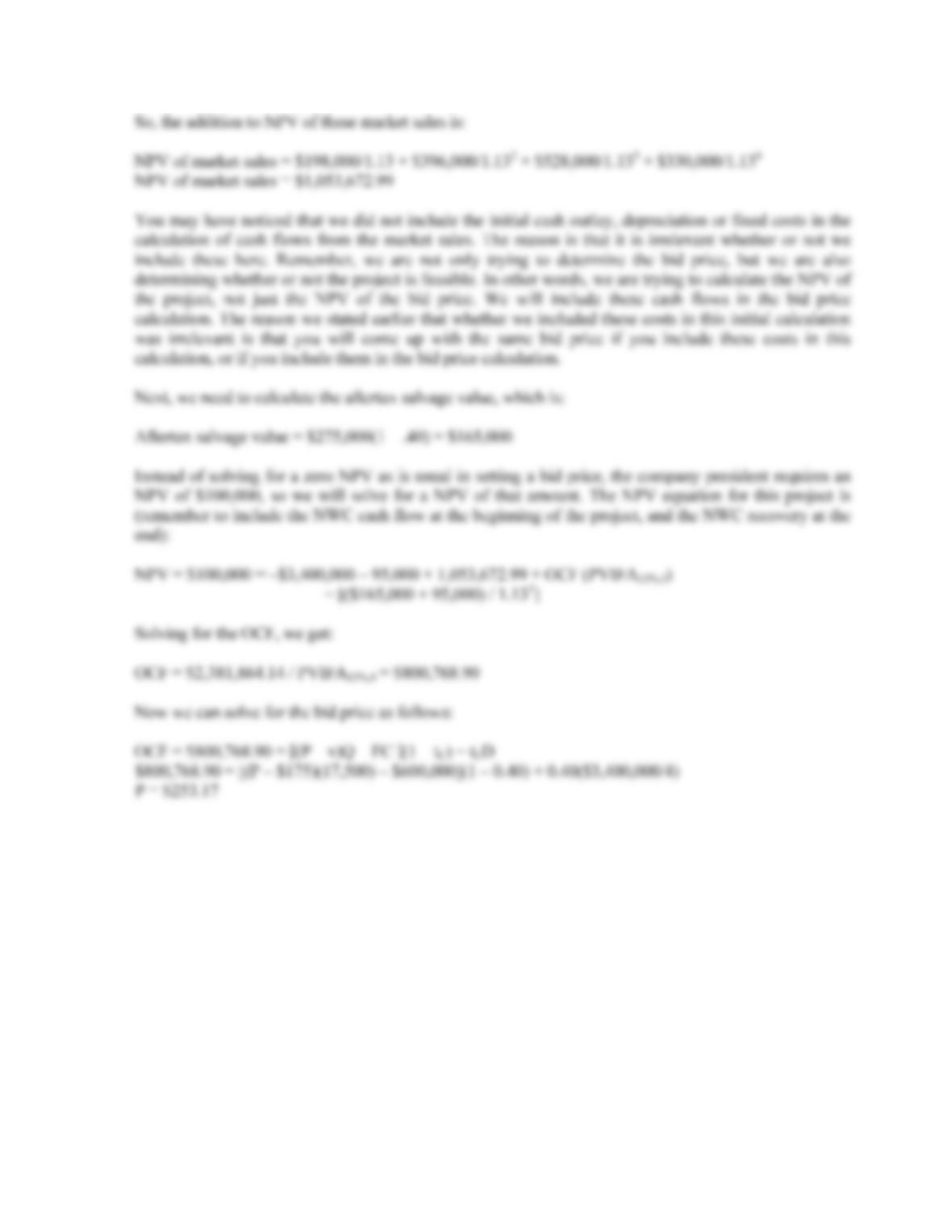B-198 SOLUTIONS
36. a. Since the two computers have unequal lives, the correct method to analyze the decision is the
EAC. We will begin with the EAC of the new computer. Using the depreciation tax shield
approach, the OCF for the new computer system is:
OCF = (\$145,000)(1 – .38) + (\$780,000 / 5)(.38) = \$149,180
Notice that the costs are positive, which represents a cash inflow. The costs are positive in this
case since the new computer will generate a cost savings. The only initial cash flow for the new
computer is cost of \$780,000. We next need to calculate the aftertax salvage value, which is:
Aftertax salvage value = \$150,000(1 – .38) = \$93,000
Now we can calculate the NPV of the new computer as:
NPV = –\$780,000 + \$149,180(PVIFA12%,5) + \$93,000 / 1.125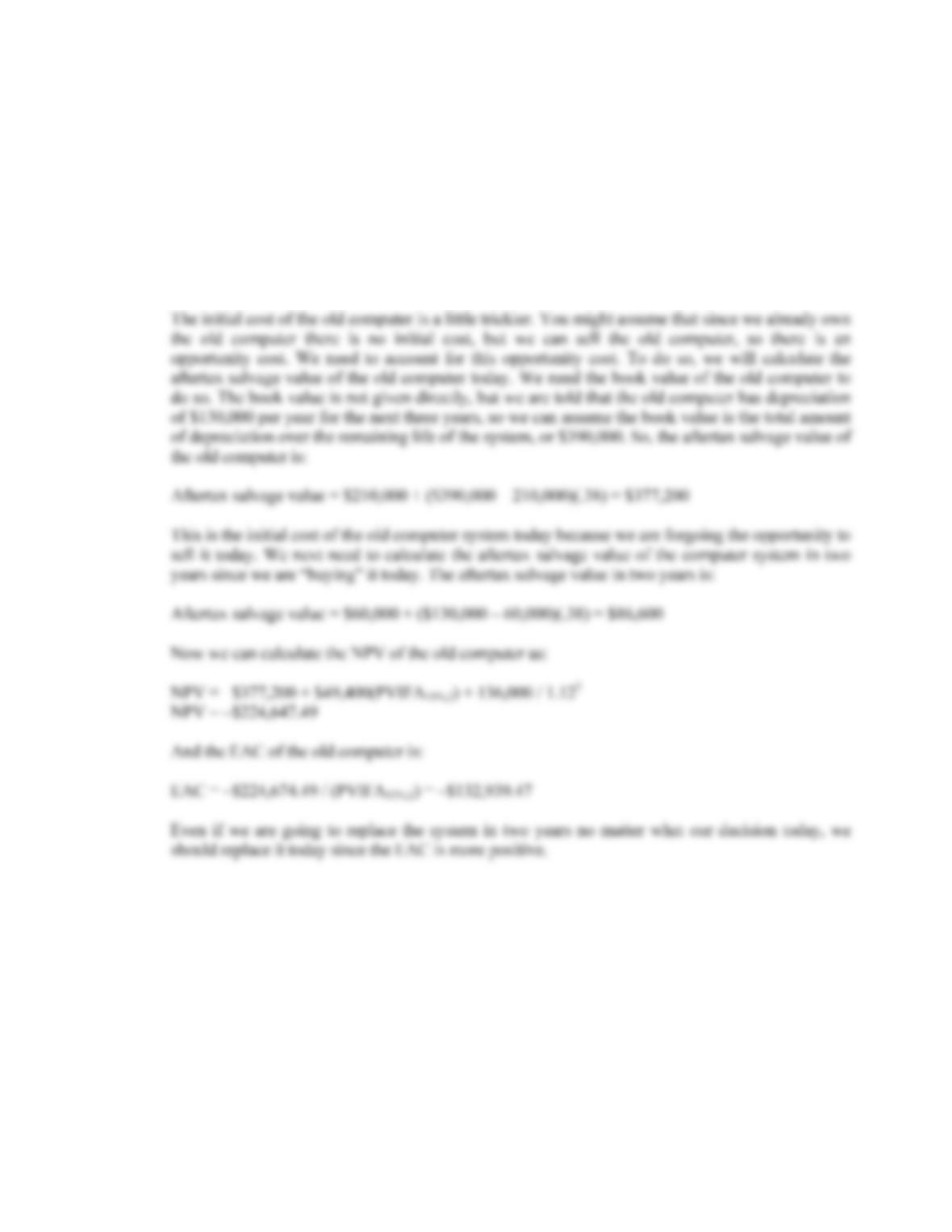CHAPTER 10 B-199
NPV = –\$189,468.79
And the EAC of the new computer is:
EAC = –\$189,468.79 / (PVIFA12%,5) = –\$52,560.49
Analyzing the old computer, the only OCF is the depreciation tax shield, so:
OCF = \$130,000(.38) = \$49,400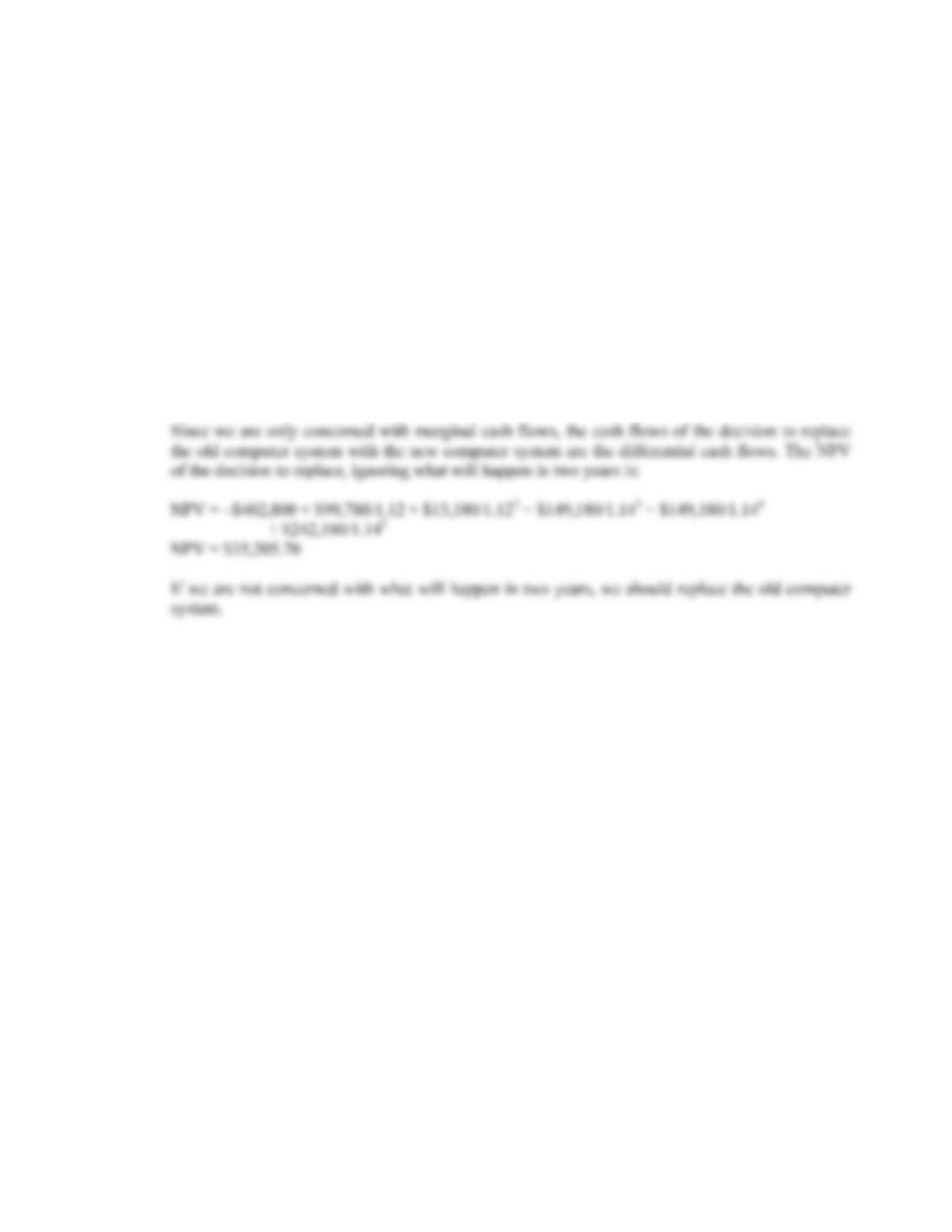B-200 SOLUTIONS
b. If we are only concerned with whether or not to replace the machine now, and are not worrying
about what will happen in two years, the correct analysis is NPV. To calculate the NPV of the
decision on the computer system now, we need the difference in the total cash flows of the old
computer system and the new computer system. From our previous calculations, we can say the
cash flows for each computer system are:
t New computer Old computer Difference
0 –\$780,000 –\$377,200 –\$402,800
1 149,180 49,400 99,780
2 149,180 136,000 13,180
3 149,180 0 149,180
4 149,180 0 149,180
5 242,180 0 242,180

## Trusted by Thousands ofStudents

Here are what students say about us.